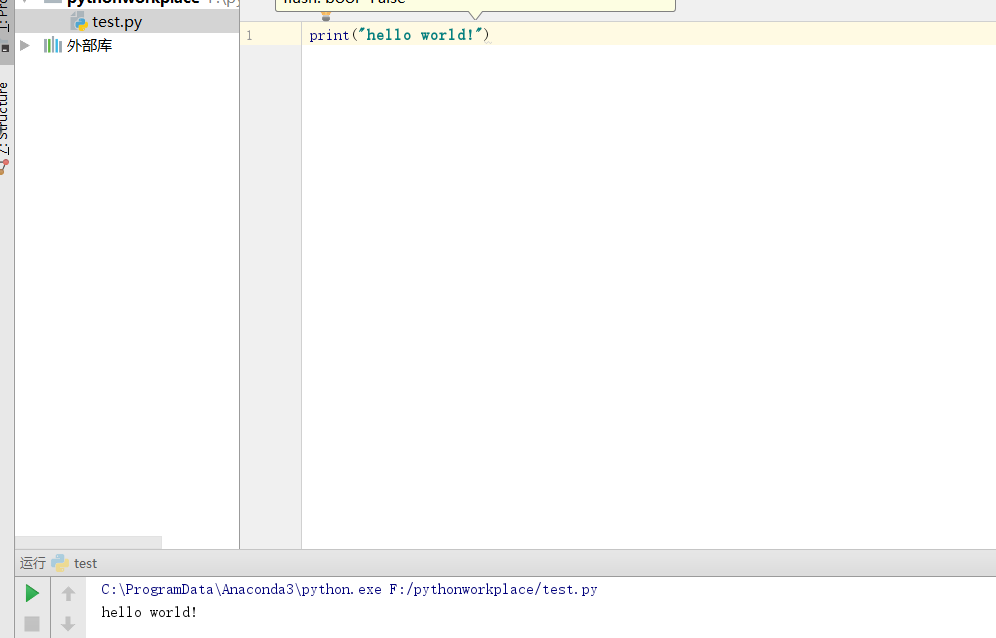# 一、anaconda的安装及简单使用

Anaconda是一个用于科学计算的Python发行版，支持 Linux, Mac, Windows系统，提供了包管理与环境管理的功能，可以很方便地解决多版本python并存、切换以及各种第三方包安装问题。Anaconda利用工具/命令conda来进行package和environment的管理，并且已经包含了Python和相关的配套工具方式

## 1.下载anaconda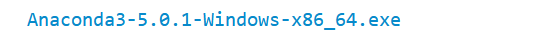## 2.安装anaconda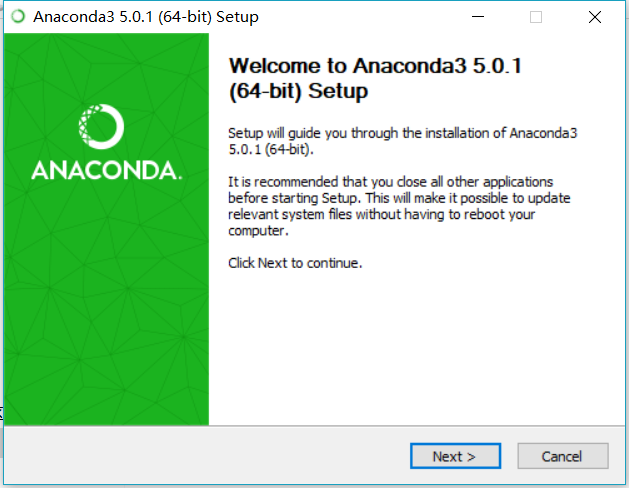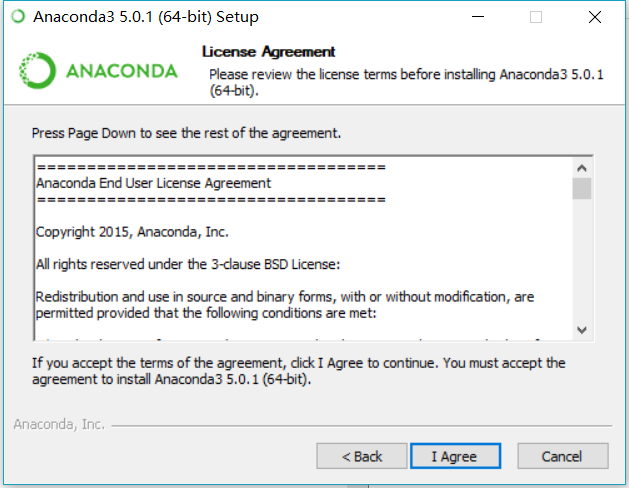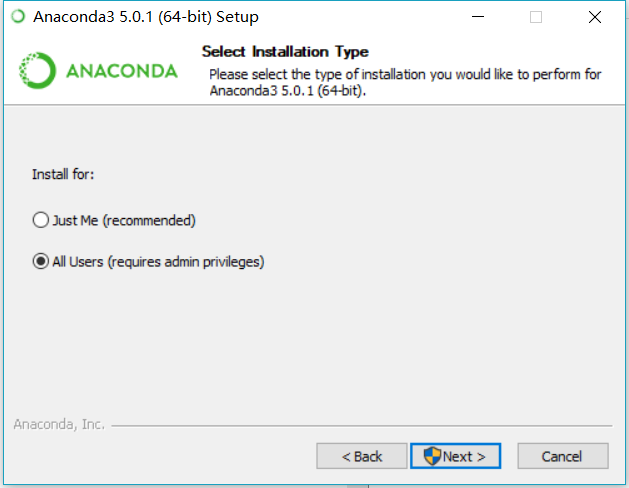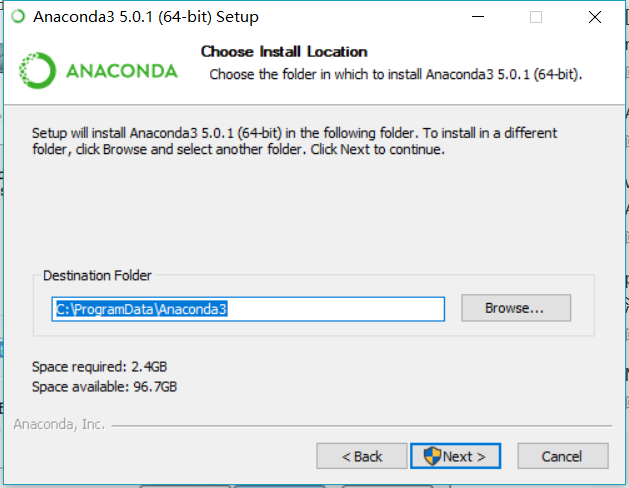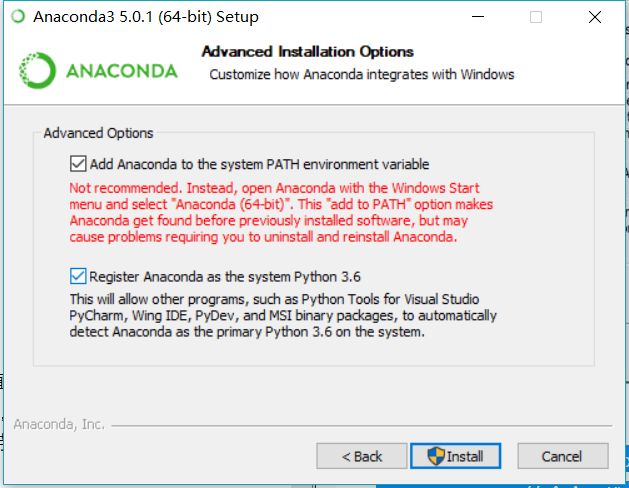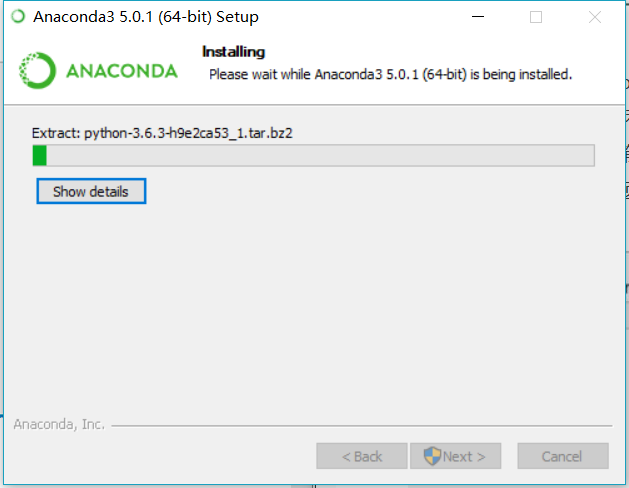## 3.配置anaconda

``````conda config --add channels https://mirrors.tuna.tsinghua.edu.cn/anaconda/pkgs/free/

conda config --set show_channel_urls yes``````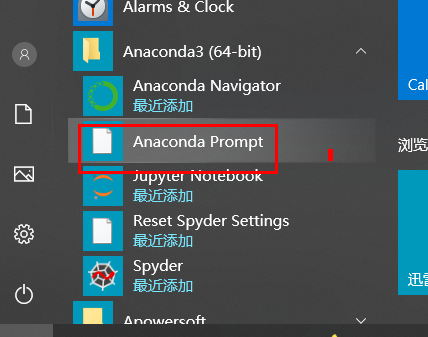### 3.1Anaconda Prompt的简单使用

``````conda config --add channels https://mirrors.tuna.tsinghua.edu.cn/anaconda/pkgs/free/

conda config --set show_channel_urls yes``````

Anaconda Prompt继续运行：

conda list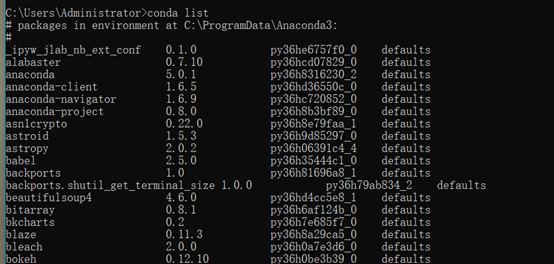conda info –envs   找到anaconda安装位置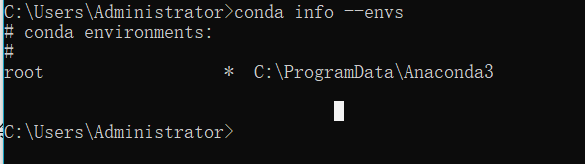conda install <包名> 安装指定包

conda remove <包名> 移除指定包

conda update <包名> 更新指定包

### 3.2初用Anaconda的spyder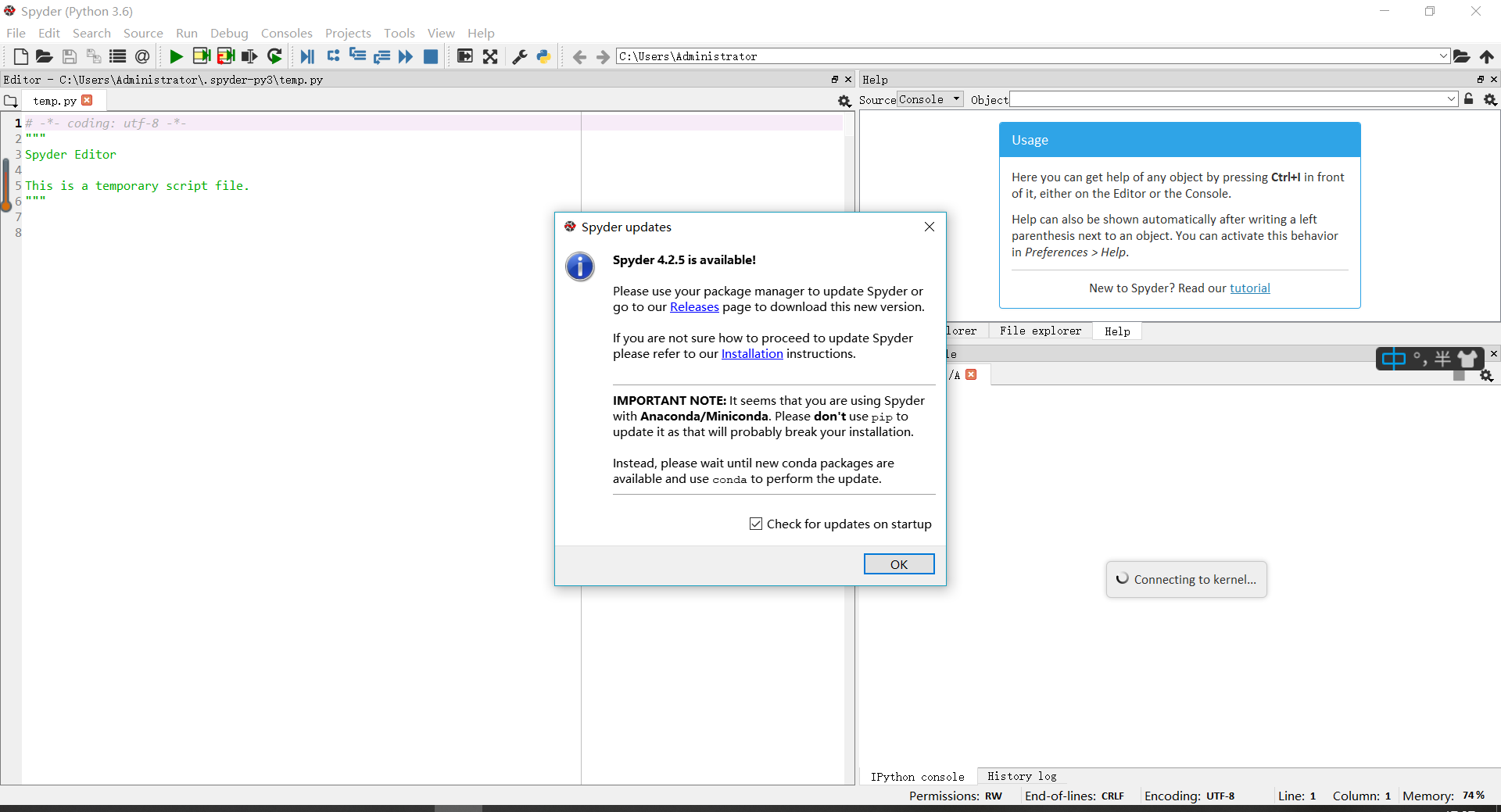``````from mpl_toolkits.mplot3d import axes3d
import matplotlib.pyplot as plt
from matplotlib import cm

fig = plt.figure()
ax = fig.gca(projection='3d')
X, Y, Z = axes3d.get_test_data(0.05)
ax.plot_surface(X, Y, Z, rstride=8,

cstride=8, alpha=0.3)
cset = ax.contour(X, Y, Z, zdir='z',

offset=-100, cmap=cm.coolwarm)
cset = ax.contour(X, Y, Z, zdir='x',

offset=-40, cmap=cm.coolwarm)
cset = ax.contour(X, Y, Z, zdir='y',

offset=40, cmap=cm.coolwarm)

ax.set_xlabel('X')
ax.set_xlim(-40, 40)
ax.set_ylabel('Y')
ax.set_ylim(-40, 40)
ax.set_zlabel('Z')
ax.set_zlim(-100, 100)

plt.show()
``````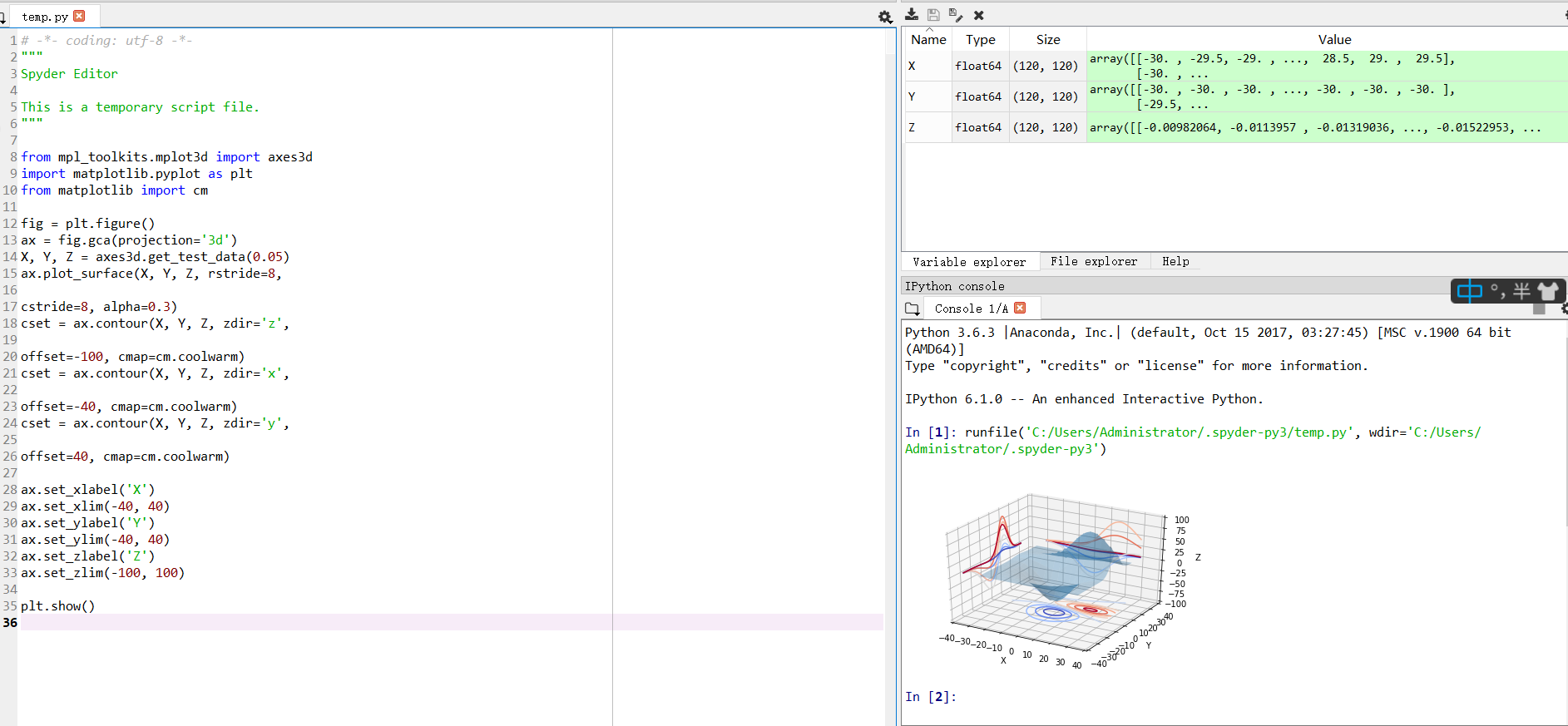anaconda的安装及简单应用到此结束

# 三、在pycharm下配置anaconda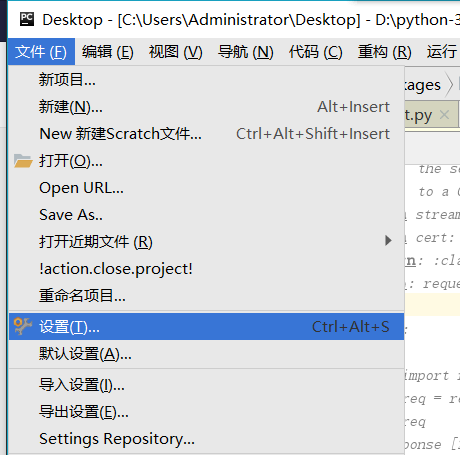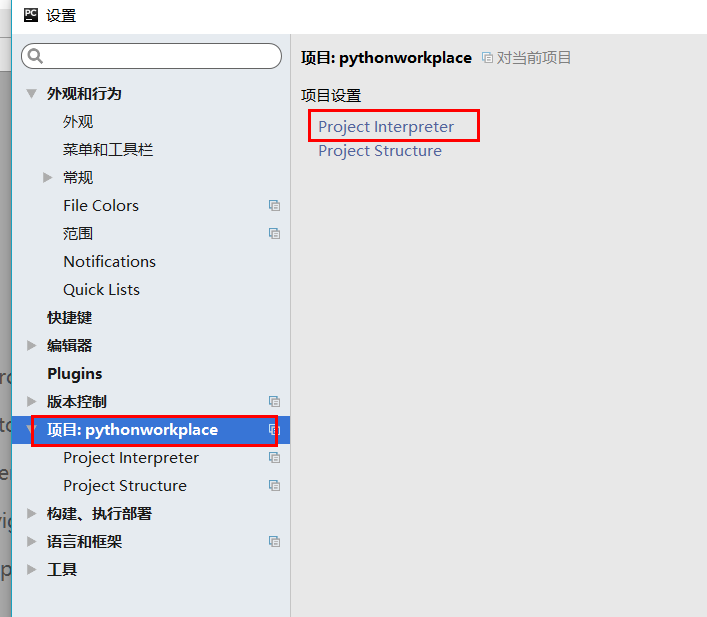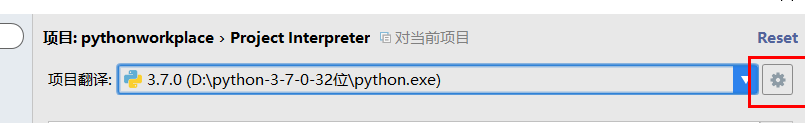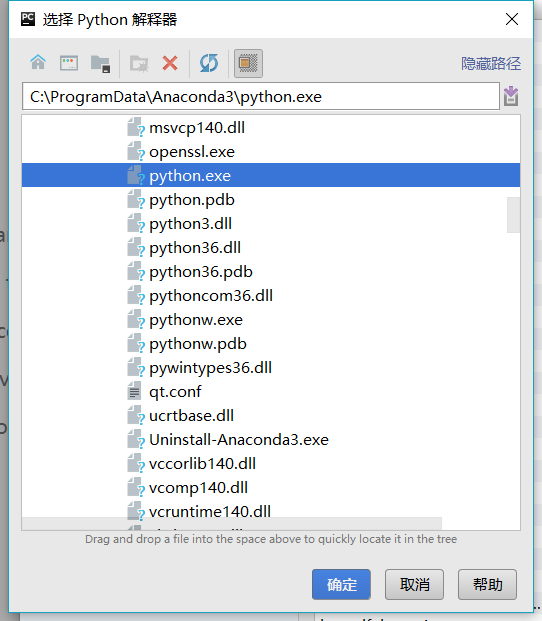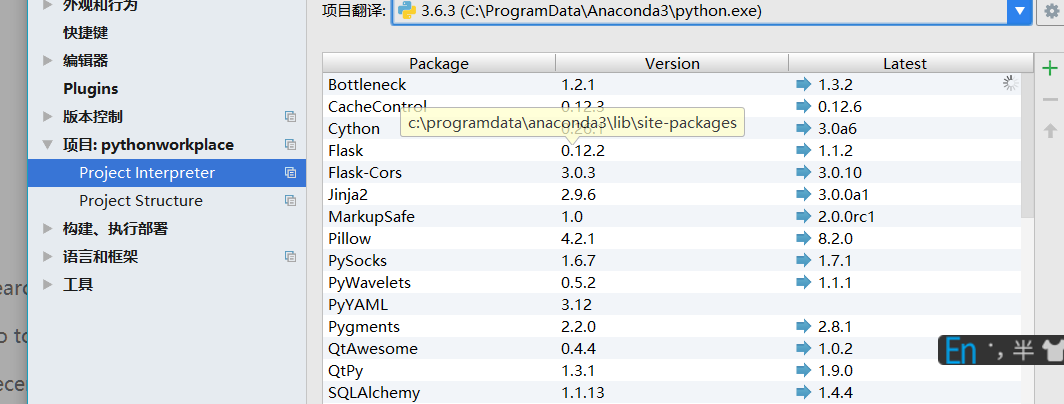ps：初次导入anaconda进程序，系统会比较慢

# 四、测试安装配置成功